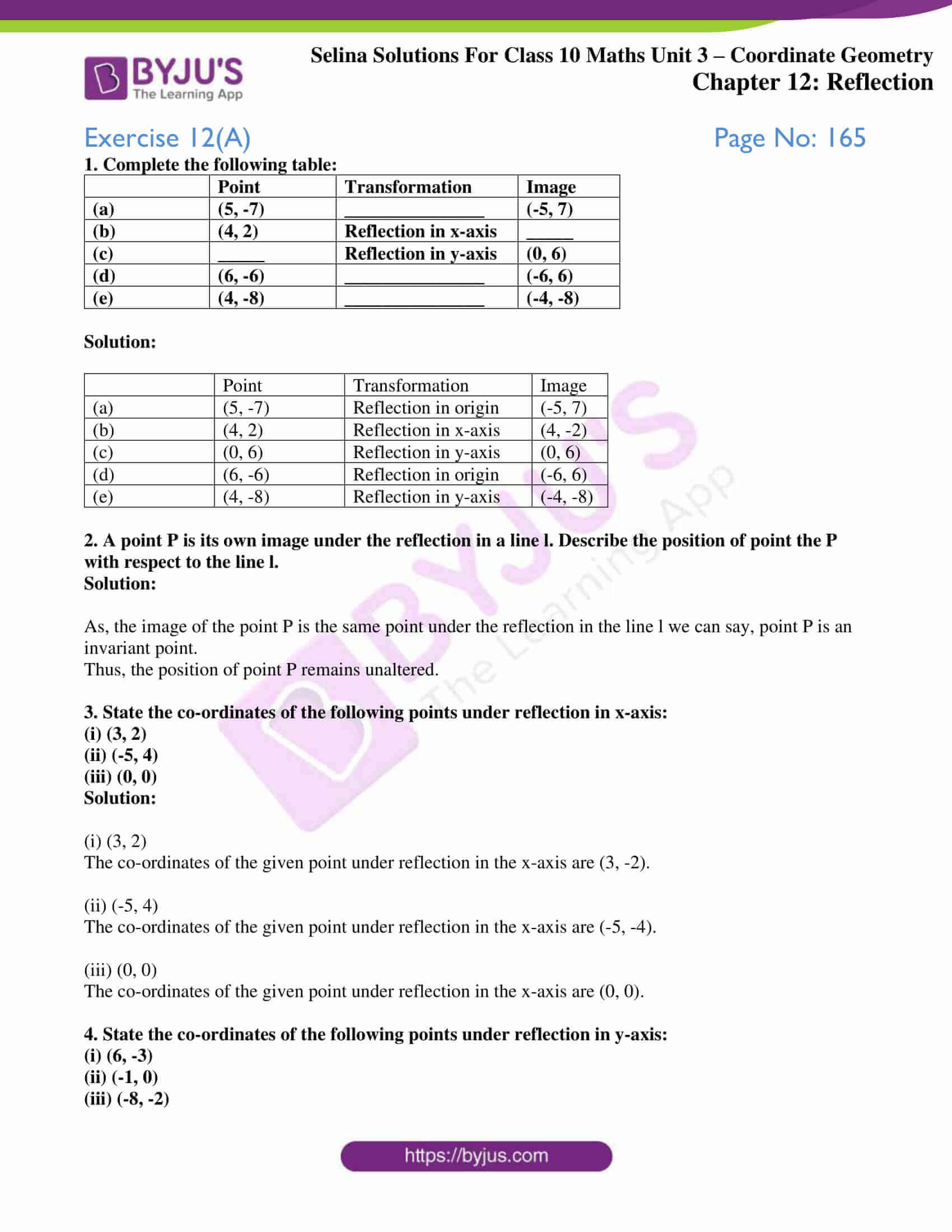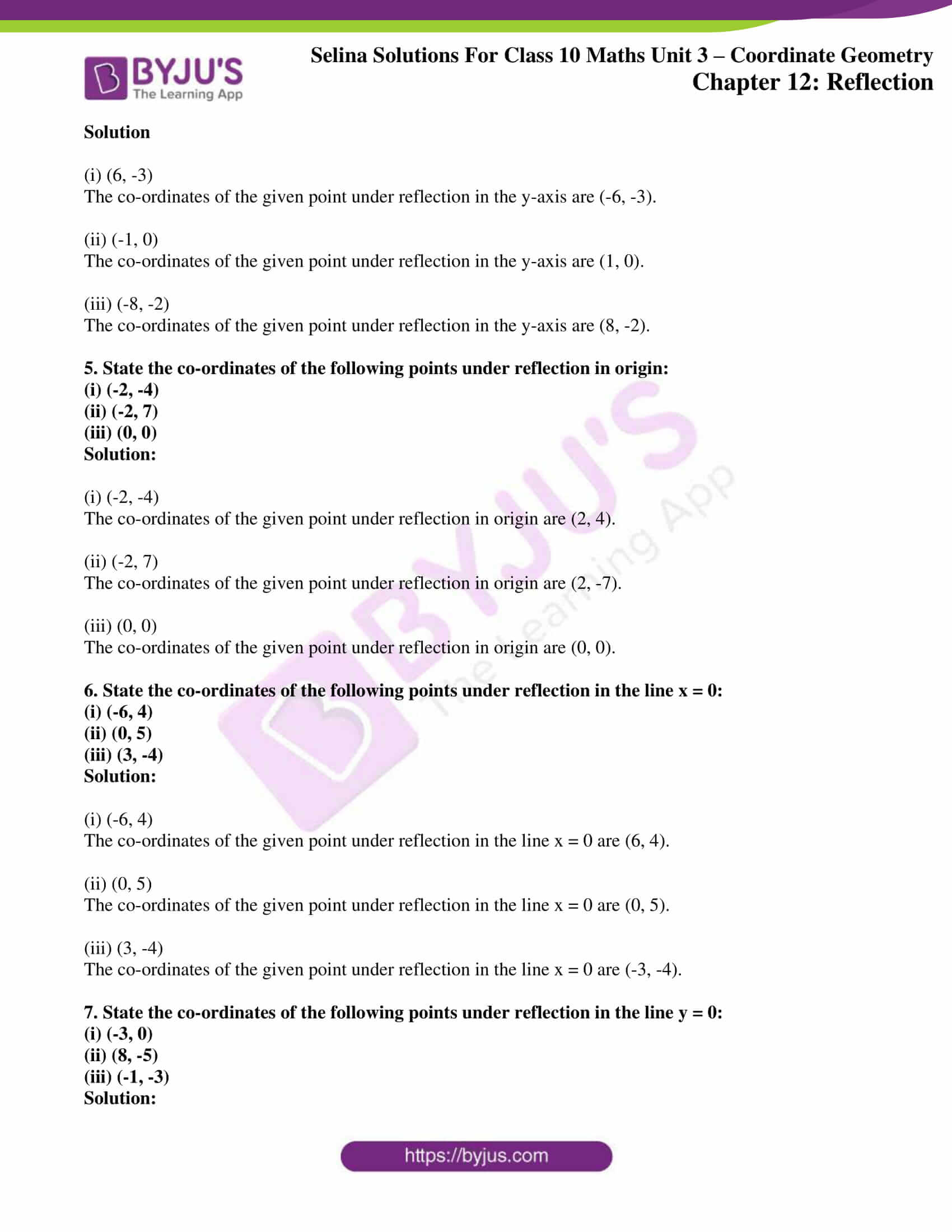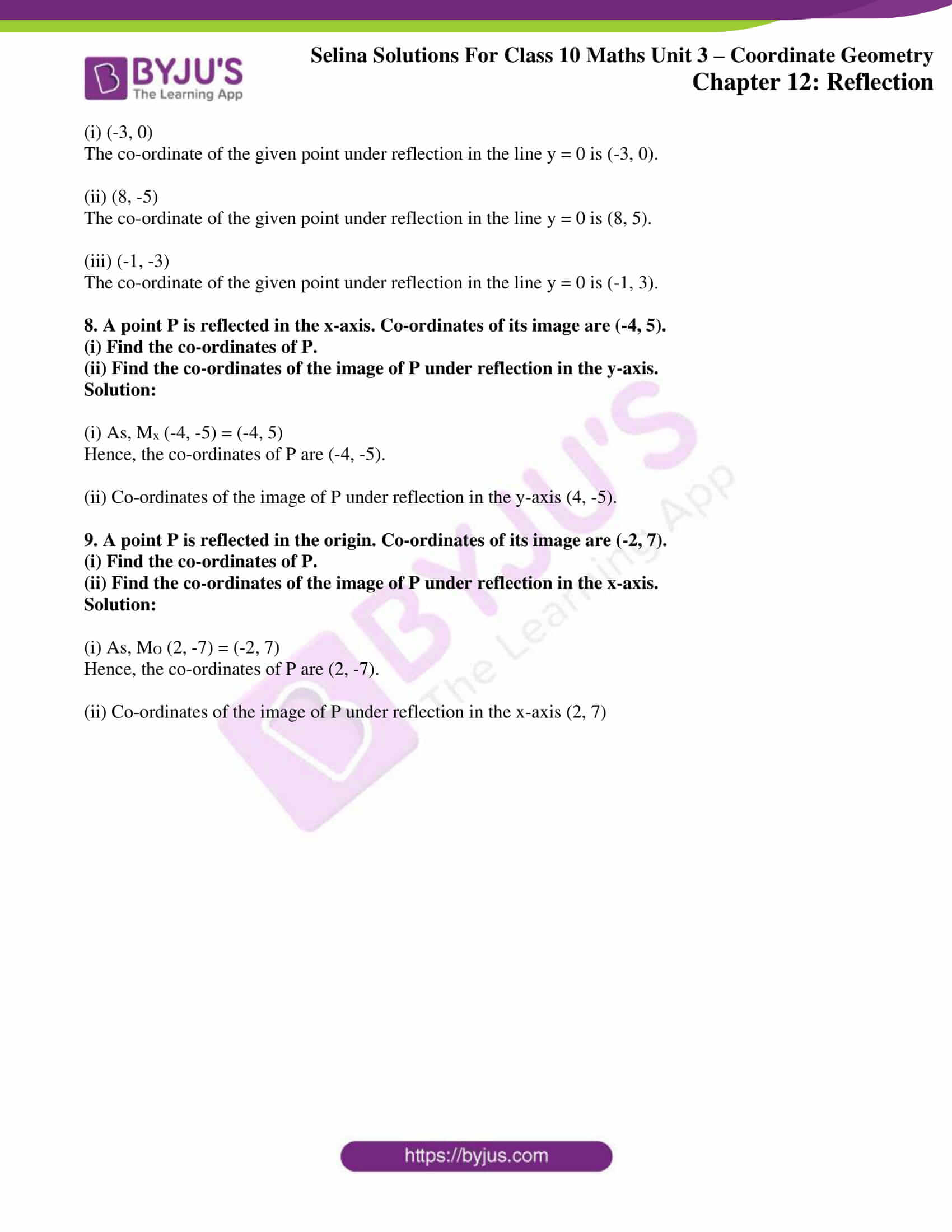# Selina Solutions Concise Maths Class 10 Chapter 12 Reflection Exercise 12(A)

The concept of co-ordinate axes, co-ordinates, the reflection of a point in origin and coordinate axes and invariant point are explained and tested in this exercise. Students having any doubts regarding solving problems of this Chapter can refer to Selina Solutions for Class 10 Maths. These solutions are developed by a set of subject matter experts having vast experience in the academic field. Further, the step by step answers to this exercise are available in the Selina Solutions Concise Maths Class 10 Chapter 12 Reflection Exercise 12(A) PDF in the links given below.

## Selina Solutions Concise Maths Class 10 Chapter 12 Reflection Exercise 12(A) Download PDF### Access another exercise of Selina Solutions Concise Maths Class 10 Chapter 12 Reflection

Exercise 12(B) Solutions

### Access Selina Solutions Concise Maths Class 10 Chapter 12 Reflection Exercise 12(A)

Exercise 12(A) Page No: 165

1. Complete the following table:

 Point Transformation Image (a) (5, -7) _______________ (-5, 7) (b) (4, 2) Reflection in x-axis _____ (c) _____ Reflection in y-axis (0, 6) (d) (6, -6) _______________ (-6, 6) (e) (4, -8) _______________ (-4, -8)

Solution:

 Point Transformation Image (a) (5, -7) Reflection in origin (-5, 7) (b) (4, 2) Reflection in x-axis (4, -2) (c) (0, 6) Reflection in y-axis (0, 6) (d) (6, -6) Reflection in origin (-6, 6) (e) (4, -8) Reflection in y-axis (-4, -8)

2. A point P is its own image under the reflection in a line l. Describe the position of point the P with respect to the line l.

Solution:

As, the image of the point P is the same point under the reflection in the line l we can say, point P is an invariant point.

Thus, the position of point P remains unaltered.

3. State the co-ordinates of the following points under reflection in x-axis:

(i) (3, 2)

(ii) (-5, 4)

(iii) (0, 0)

Solution:

(i) (3, 2)

The co-ordinates of the given point under reflection in the x-axis are (3, -2).

(ii) (-5, 4)

The co-ordinates of the given point under reflection in the x-axis are (-5, -4).

(iii) (0, 0)

The co-ordinates of the given point under reflection in the x-axis are (0, 0).

4. State the co-ordinates of the following points under reflection in y-axis:

(i) (6, -3)

(ii) (-1, 0)

(iii) (-8, -2)

Solution

(i) (6, -3)

The co-ordinates of the given point under reflection in the y-axis are (-6, -3).

(ii) (-1, 0)

The co-ordinates of the given point under reflection in the y-axis are (1, 0).

(iii) (-8, -2)

The co-ordinates of the given point under reflection in the y-axis are (8, -2).

5. State the co-ordinates of the following points under reflection in origin:

(i) (-2, -4)

(ii) (-2, 7)

(iii) (0, 0)

Solution:

(i) (-2, -4)

The co-ordinates of the given point under reflection in origin are (2, 4).

(ii) (-2, 7)

The co-ordinates of the given point under reflection in origin are (2, -7).

(iii) (0, 0)

The co-ordinates of the given point under reflection in origin are (0, 0).

6. State the co-ordinates of the following points under reflection in the line x = 0:

(i) (-6, 4)

(ii) (0, 5)

(iii) (3, -4)

Solution:

(i) (-6, 4)

The co-ordinates of the given point under reflection in the line x = 0 are (6, 4).

(ii) (0, 5)

The co-ordinates of the given point under reflection in the line x = 0 are (0, 5).

(iii) (3, -4)

The co-ordinates of the given point under reflection in the line x = 0 are (-3, -4).

7. State the co-ordinates of the following points under reflection in the line y = 0:

(i) (-3, 0)

(ii) (8, -5)

(iii) (-1, -3)

Solution:

(i) (-3, 0)

The co-ordinate of the given point under reflection in the line y = 0 is (-3, 0).

(ii) (8, -5)

The co-ordinate of the given point under reflection in the line y = 0 is (8, 5).

(iii) (-1, -3)

The co-ordinate of the given point under reflection in the line y = 0 is (-1, 3).

8. A point P is reflected in the x-axis. Co-ordinates of its image are (-4, 5).

(i) Find the co-ordinates of P.

(ii) Find the co-ordinates of the image of P under reflection in the y-axis.

Solution:

(i) As, Mx (-4, -5) = (-4, 5)

Hence, the co-ordinates of P are (-4, -5).

(ii) Co-ordinates of the image of P under reflection in the y-axis (4, -5).

9. A point P is reflected in the origin. Co-ordinates of its image are (-2, 7).

(i) Find the co-ordinates of P.

(ii) Find the co-ordinates of the image of P under reflection in the x-axis.

Solution:

(i) As, MO (2, -7) = (-2, 7)

Hence, the co-ordinates of P are (2, -7).

(ii) Co-ordinates of the image of P under reflection in the x-axis (2, 7)